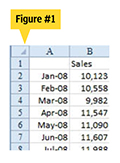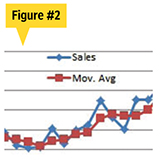Technology

# An Average Solution

How to create a prediction of sales in Excel using a moving average and a trend line.Q: I have 36 months of sales data and need to create a prediction of sales. How can I calculate a 3-month moving average and a trend line based on that average?

A: You need 2 months of history before you can begin calculating a 3-month moving average. When you have a data set similar to Figure 1, for example, follow these steps:

1. In cell C4, enter the formula =AVERAGE(B2:B4). Note that when you enter this formula, Excel will be concerned because the formula will ignore similar data in cell B5. In this case, you are smarter than Excel, so you can use the Caution (exclamation point) dropdown to tell Excel to ignore the error.2. Double-click the fill handle in C4 to copy the formula down to the rest of your data set.

Moving averages are good if the underlying data has spikes in the sales. It is difficult for an automatic system to predict spikes. A moving average smooths these spikes out of the system. A forecast based on such an average line may be more accurate than a forecast based on the original data. Figure 2 illustrates the 3-month moving average and a forecastable trend.

If you would like to submit a question to Bill “MrExcel” Jelen, go to CFO’s Spreadsheet Community Center at www.cfo.com/spreadsheets.